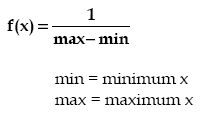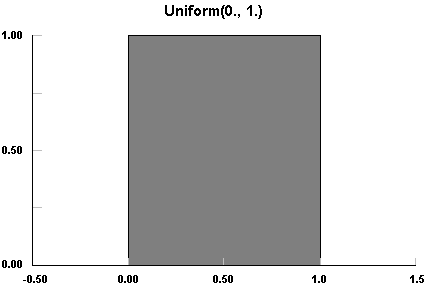AnyLogic

# uniformThe Uniform distribution is a continuous distribution bounded on both sides, i.e. the sample lays in the interval [min,max). The probability density does not depend on the value of x. It is a special case of the Beta distribution. It is frequently called rectangular distribution (see Johnson et al).

The Uniform distribution is used to represent a random variable with constant likelihood of being in any small interval between min and max. Note that the probability of max value is 0; the max point never occurs.

### Sample### uniform()

Description
Generates a random value uniformly distributed on the interval [0,1), the upper bound is not included.
Result
Type Description
double the generated sample

### uniform(java.util.Random r)

Description
Generates a random value uniformly distributed on the interval [0,1), using the specified random number generator.
Parameters
Name Type Description
r java.util.Random the random number generator
Result
Type Description
double the generated sample

### uniform(double max)

Description
Generates a sample of the Uniform distribution on the interval [0, max). Is equivalent to uniform(0, max). For more details see uniform(double,double).
Parameters
Name Type Description
max double the maximum x value
Result
Type Description
double the generated sample

### uniform(double min, double max)

Description
Generates a sample of the Uniform distribution on the interval [min, max).
Parameters
Name Type Description
min double the minimum x value
max double the maximum x value
Result
Type Description
double the generated sample

### uniform(double min, double max, java.util.Random r)

Description
Generates a sample of the Uniform distribution on the interval [min, max) using the specified random number generator. For more details see uniform(double,double).
Parameters
Name Type Description
min double the minimum x value
max double the maximum x value
r java.util.Random the random number generator
Result
Type Description
double the generated sample

This document includes content from the Stat::Fit User's Manual. Copyright 2016 Geer Mountain Software Corp.